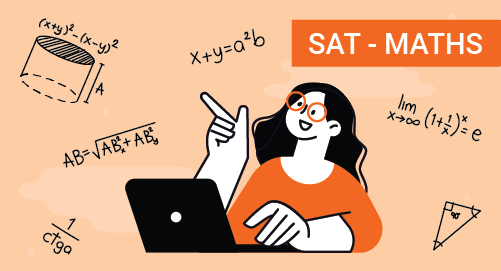#### Need Help?

Get in touch with usSAT

# SAT Mathematics

Maths

Scholastic Aptitude Test or SAT is conducted by College Baord. Most of the universities and colleges provide admissions based onn the SAT score. SaT has upgraded to Digital SAT to adapt the changing needs of students and educators. SAT focuses on evidence-based reading, writing, problems solving skills and data analysis. It can be attempted multipled times in an year.

##### Course Duration
•NA40 Sessions
•30 mins per session
##### Tutor## What you'll learn

• 1

Linear Equations in 1-variable and 2-variables, linear functions and quadratic functions.

• 2

Graphical representation of Algebraic Expressions

• 3

Ratio, proportion, rate and percentage

• 4

Units, Measurement and unit conversion

• 5

Probability and Two-way Frequency Tables

• 6

Data collection and Representation

• 7

• 8

3D shapes and Solid Geometry

• 9

Lines, Angles and Triangles

• 10

Cicles and basic concepts of circles

• 11

Complex Numbers

## Skills you'll learnCreate and solve linear equations, and inequalities in 1 variable and 2 variablesRelation between Graphical representation and Algebraic representationSolve problems using ratios, proportions, rate, and percentageMeasurement of different quantities, their units and unit conversionUsing two-way frequency tables and calculate probabilityWriting Polynomials and basic operations on polynomialsSimplifying and solving radical functions and rational functionsUnderstand congruency and similarity. Apply for lines, angles and trianglesUnderstand trigonometric ratios and use trigonometric functions to measure anglesUnderstand the concept of circles, arc, chord length, area of sectors and anglesSimplify Complex Numbers and Basic Operations of Complex Numbers